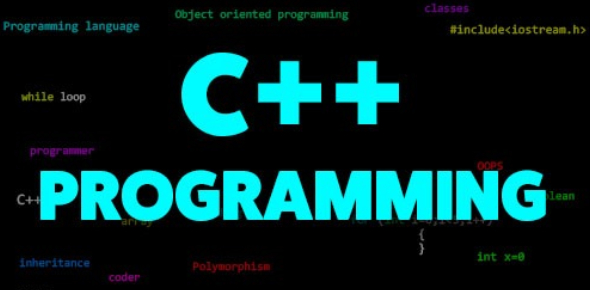# C Plus Plus Programming Quiz For Beginners

38 Questions | Attempts: 10348
ShareSettingsC++ is one of the common programming languages that most programmers are expected to have a good understanding of. Here is a C Plus Plus Programming Quiz For Beginners. Try this quiz and see if you can answer all the questions correctly or not. As you continue your journey with C++, it is important to see if you already understand the basics and this quiz will help you do just that. Give it a shot and be a step closer to being perfect when it comes to this language.

• 1.
The notation of logical NOT operator in a C++ program is
• A.

:

• B.

;

• C.

None of the Above

• D.

!

• 2.
For the class exforsys defined as below: class exforsys() { }; int main() { exforsys a; } Which of the following is TRUE?
• A.

No default constructor is provided by the compiler

• B.

The compiler provides the default constructor

• C.

The compiler generates error

• D.

None of the Above

• 3.
The notation of ternary operator is
• A.

&

• B.

?:

• C.

~

• D.

%

• 4.
When the object of the first class is instantiated then which of the following is called?
• A.

Destructor

• B.

Constructor

• C.

Both A and B

• D.

None of the Above

• 5.
Which of the following denotes the C++ looping statement?
• A.

Do-while

• B.

For

• C.

Both A and B

• 6.
Which of the following can be used to initialize a newly declared variable from an existing variable?
• A.

Virtual Function

• B.

Namespaces

• C.

Copy constructor

• D.

None of the Above

• 7.
A template can be instantiated by
• A.

Explicit Instantiation

• B.

Implicit instantiation

• C.

Both A and B

• D.

None of the Above

• 8.
The minimum number of times a do loop would definitely get executed is:
• A.

0

• B.

1

• C.

Infinity

• D.

Could not be predicted

• 9.
Strict parameter type checking is followed by which of these?
• A.

Inline

• B.

Macros

• C.

Both A and B

• D.

None of the Above

• 10.
The variables that can be used only within the function in which it is declared is called as
• A.

Global Variables

• B.

Local Variables

• C.

Both A and B

• D.

None of the Above

• 11.
How many values can be returned by a C++ function?
• A.

1

• B.

Infinity

• C.

0

• D.

None of the Above

• 12.
If a function in C++ does not return a value then its return type is denoted as
• A.

Float

• B.

Void

• C.

Int

• D.

None of the Above

• 13.
The variables that are used to represent individual array element in an array is called as
• A.

Pointer Variable

• B.

Subscripted variable

• C.

Index Variable

• D.

Real Variable

• 14.
The array exforsys can be declared using pointers as
• A.

% exforsys[]

• B.

& exforsys[]

• C.

* exforsys[]

• D.

@ exforsys[]

• 15.
What is the notation used to place block of statements in a looping structure in C++?
• A.

% %

• B.

( )

• C.

{ }

• D.

None of the Above

• 16.
Index of an array starts from
• A.

One

• B.

Zero

• C.

Two

• D.

None of the Above

• 17.
The variable that contains address of another variable is called as
• A.

Pointer

• B.

Arrays

• C.

Unions

• D.

None of the Above

• 18.
The operator that denotes address of a variable in C++ program is
• A.

*

• B.

%

• C.

\$

• D.

&

• 19.
The notation of member access operator in structures is
• A.

&

• B.

.

• C.

\$

• D.

*

• 20.
One argument constructor is also called as
• A.

Single constructor

• B.

Copy constructor

• C.

Both A and B

• D.

None of the Above

• 21.
The other name for external variables in C++ is
• A.

Static variables

• B.

Register variables

• C.

Global variables

• D.

None of the Above

• 22.
What method is used for writing characters in a C++ program?
• A.

Put

• B.

Get

• C.

Write

• D.

None of the Above

• 23.
What will be the return type for a function named as exforsys declared as int exforsys (float x, double y)?
• A.

Double

• B.

Int

• C.

Float

• D.

None of the Above

• 24.
Which of the following option denotes the newline character in the C++ program?
• A.

'\n'

• B.

'/n'

• C.

'nc'

• D.

'/nc'

• 25.
Which of the following is used to group a set of global classes, objects and functions under a name?
• A.

Area named

• B.

Namearea

• C.

Namespaces

• D.

None of the above

## Related TopicsBack to top
×

Wait!
Here's an interesting quiz for you.